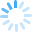# What Are The Types Of Numbers? Crossword Puzzle00: 00
• Play Again
•
•natural number:the counting number which start from1 to infinite.\r\nwhole number: the counting number which start from 0 to infinite.\r\ninteger: the positive number ,negative number zero is know as.\r\nrational number:the number which can be expressed in p\\q form where q is not zero.\r\nreal nuimber: all rational number irrational number are together called.....\r\npolynomial:an algebraic expression in which the variables involved have only one non-negative integral exponents.\r\nmonomials: polynomials having one term.\r\nbinomials: polynomials having two term.\r\ntrinomials:polynomials having only three term.